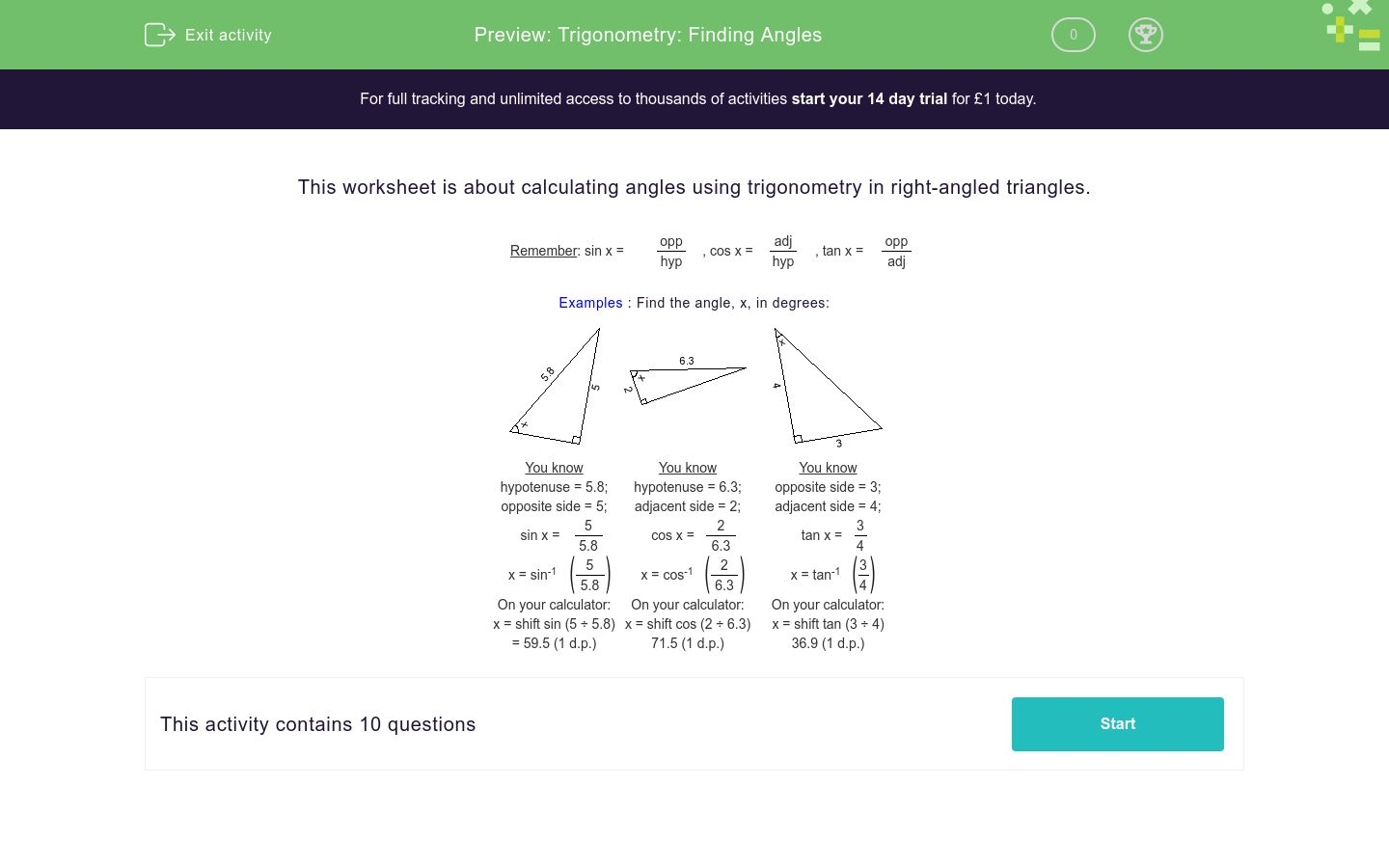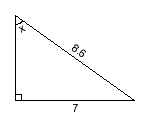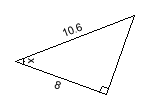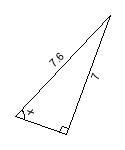# Trigonometry: Finding Angles

In this worksheet, students use trigonometry to calculate angles in right-angled triangles.Key stage:  KS 4

Curriculum topic:  Geometry and Measures

Difficulty level:### QUESTION 1 of 10

This worksheet is about calculating angles using trigonometry in right-angled triangles.

 Remember: sin x = opp , cos x = adj , tan x = opp hyp hyp adj

Examples : Find the angle, x, in degrees:You know
hypotenuse = 5.8;
opposite side = 5;
 sin x = 5 5.8
 x = sin-155.8
On your calculator:
x = shift sin (5 ÷ 5.8)
= 59.5 (1 d.p.)
You know
hypotenuse = 6.3;
adjacent side = 2;
 cos x = 2 6.3
 x = cos-126.3
On your calculator:
x = shift cos (2 ÷ 6.3)
71.5 (1 d.p.)
You know
opposite side = 3;
adjacent side = 4;
 tan x = 3 4
 x = tan-134
On your calculator:
x = shift tan (3 ÷ 4)
36.9 (1 d.p.)

Find the angle marked x, in degrees, to 1 decimal place.

(Just write the number of degrees)x =

Find the angle marked x, in degrees, to 1 decimal place.x =

Find the angle marked x, in degrees, to 1 decimal place.x =

Find the angle marked x, in degrees, to 1 decimal place.x =

Find the angle marked x, in degrees, to 1 decimal place.x =

Find the angle marked x, in degrees, to 1 decimal place.x =

Find the angle marked x, in degrees, to 1 decimal place.x =

Find the angle marked x, in degrees, to 1 decimal place.x =

Find the angle marked x, in degrees, to 1 decimal place.x =

Find the angle marked x, in degrees, to 1 decimal place.x =
• Question 1

Find the angle marked x, in degrees, to 1 decimal place.

(Just write the number of degrees)x =
CORRECT ANSWER
54.5
EDDIE SAYS
x = sin-1(7/8.6)
• Question 2

Find the angle marked x, in degrees, to 1 decimal place.x =
CORRECT ANSWER
11.3
EDDIE SAYS
x = tan-1(2/10)
• Question 3

Find the angle marked x, in degrees, to 1 decimal place.x =
CORRECT ANSWER
15.9
EDDIE SAYS
x = tan-1(2/7)
• Question 4

Find the angle marked x, in degrees, to 1 decimal place.x =
CORRECT ANSWER
33.7
EDDIE SAYS
x = sin-1(4/7.2)
• Question 5

Find the angle marked x, in degrees, to 1 decimal place.x =
CORRECT ANSWER
35.5
EDDIE SAYS
x = tan-1(5/7)
• Question 6

Find the angle marked x, in degrees, to 1 decimal place.x =
CORRECT ANSWER
41.0
EDDIE SAYS
x = cos-1(8/10.6)
• Question 7

Find the angle marked x, in degrees, to 1 decimal place.x =
CORRECT ANSWER
49.5
EDDIE SAYS
x = sin-1(7/9.2)
• Question 8

Find the angle marked x, in degrees, to 1 decimal place.x =
CORRECT ANSWER
53.1
EDDIE SAYS
x = tan-1(4/3)
• Question 9

Find the angle marked x, in degrees, to 1 decimal place.x =
CORRECT ANSWER
59.5
EDDIE SAYS
x = sin-1(5/5.8)
• Question 10

Find the angle marked x, in degrees, to 1 decimal place.x =
CORRECT ANSWER
67.1
EDDIE SAYS
x = sin-1(7/7.6)
---- OR ----

Sign up for a £1 trial so you can track and measure your child's progress on this activity.

### What is EdPlace?

We're your National Curriculum aligned online education content provider helping each child succeed in English, maths and science from year 1 to GCSE. With an EdPlace account you’ll be able to track and measure progress, helping each child achieve their best. We build confidence and attainment by personalising each child’s learning at a level that suits them.

Start your £1 trial